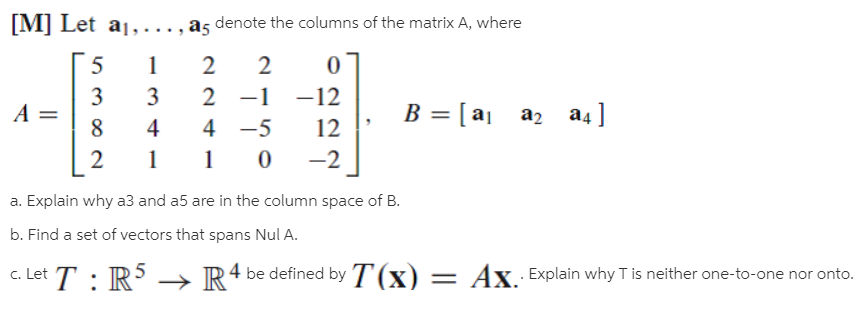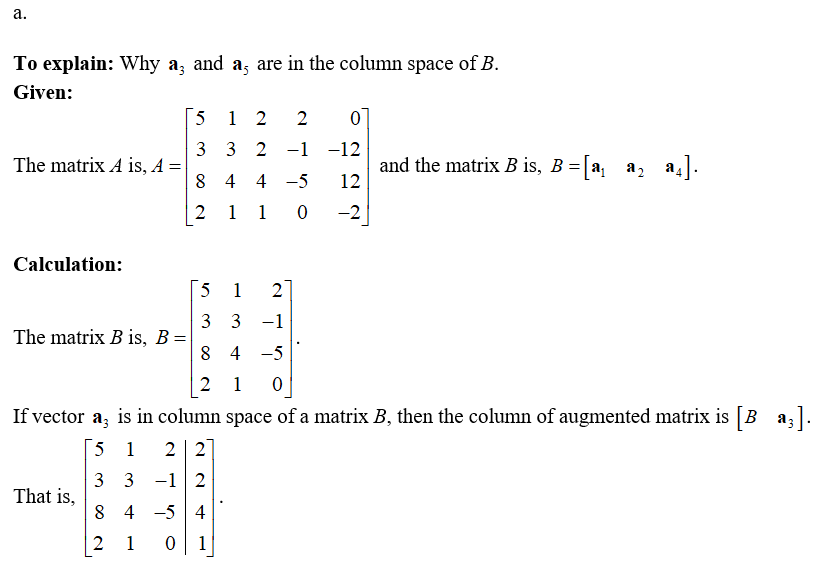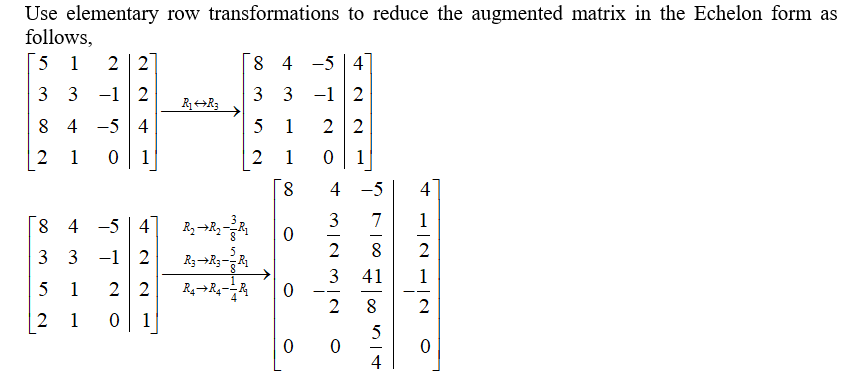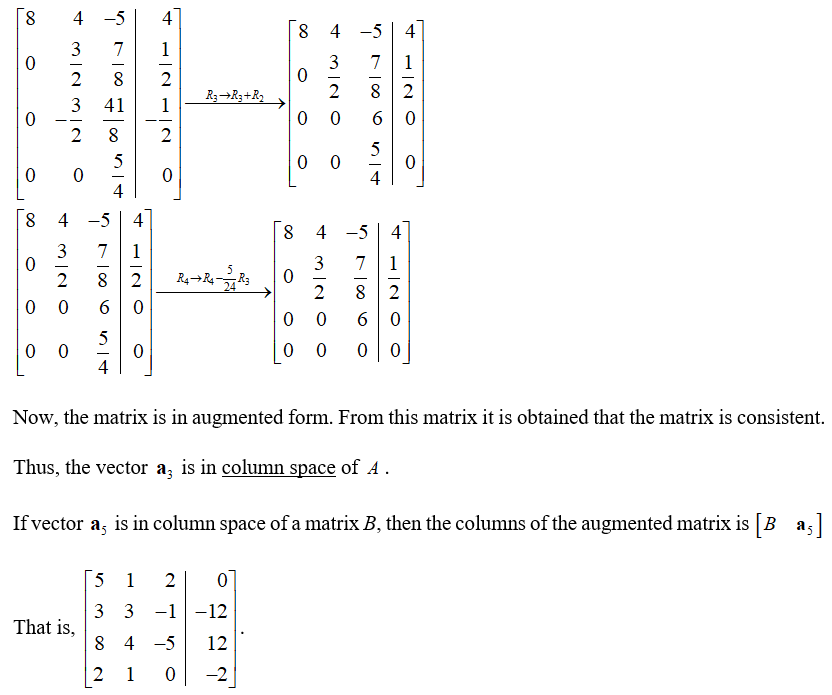# [M] Let a1,..., a5 denote the columns of the matrix A, where22332 -1-12B = [ ajaz a4]%3D44 -512-2a. Explain why a3 and a5 are in the column space of B.b. Find a set of vectors that spans Nul A.c. Let T : R4 be defined by T(x) = Ax Explain why T is neither one-to-one nor onto.

Question
21 viewshelp_outlineImage Transcriptionclose[M] Let a1,..., a5 denote the columns of the matrix A, where 2 2 3 3 2 -1 -12 B = [ aj az a4] %3D 4 4 -5 12 -2 a. Explain why a3 and a5 are in the column space of B. b. Find a set of vectors that spans Nul A. c. Let T : R 4 be defined by T(x) = Ax Explain why T is neither one-to-one nor onto. fullscreen
check_circle

Step 1Step 2Step 3...

### Want to see the full answer?

See Solution

#### Want to see this answer and more?

Solutions are written by subject experts who are available 24/7. Questions are typically answered within 1 hour.*

See Solution
*Response times may vary by subject and question.
Tagged in